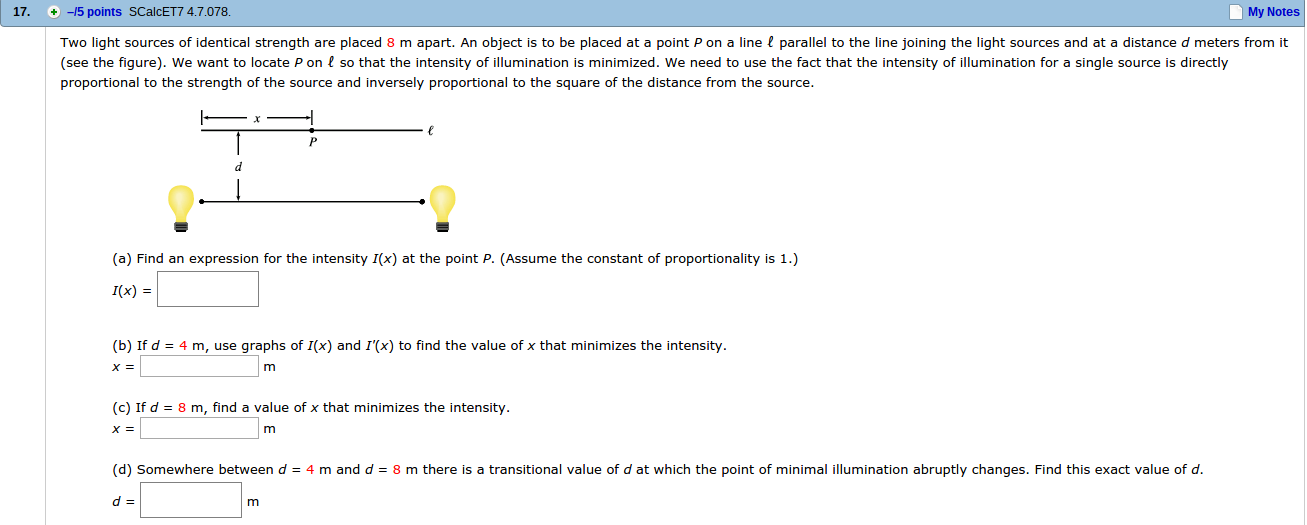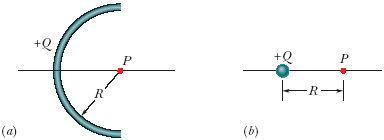Ask question

# Let C be a circle, and let P be a point not on the circle. Prove that the maximum and minimum distances from P to a point X on C occur when the line X P goes through the center of C. [Hint: Choose coordinate systems so that C is defined by x2 + y2 = r2 and P is a point (a,0) on the x-axis with a neq pm r, use calculus to find the maximum and minimum for the square of the distance. Don’t forget to pay attention to endpoints and places where a derivative might not exist.]# Let C be a circle, and let P be a point not on the circle. Prove that the maximum and minimum distances from P to a point X on C occur when the line X P goes through the center of C. [Hint: Choose coordinate systems so that C is defined by x2 + y2 = r2 and P is a point (a,0) on the x-axis with a neq pm r, use calculus to find the maximum and minimum for the square of the distance. Don’t forget to pay attention to endpoints and places where a derivative might not exist.]

Question
Alternate coordinate systemsasked 2020-12-14
Let C be a circle, and let P be a point not on the circle. Prove that the maximum and minimum distances from P to a point X on C occur when the line X P goes through the center of C. [Hint: Choose coordinate systems so that C is defined by
$$x2 + y2 = r2$$ and P is a point (a,0)
on the x-axis with a $$\neq \pm r,$$ use calculus to find the maximum and minimum for the square of the distance. Don’t forget to pay attention to endpoints and places where a derivative might not exist.]

## Answers (1)2020-12-15
The equation for C is $$x^{2} + y^{2} = r^{2}$$ and P is a point (a, 0)
on the x-axis with a $$\neq \pm r.$$
The point on the circle C is(x, y).
Obtain the distance between (x, y) and (a, 0) as follows:
$$d =\sqrt{(x - a)^2 + (y - 0)^2}$$
$$=\sqrt{(x-a)^{2}+y^{2}}$$
Now, obtain the partial derivatives as follows,
$$d_{x} = 0$$
$$\frac{2(x-a)}{2\sqrt{(x-a)^{2}+y^{2}}}=0$$
$$x = a$$
$$d_{y} = 0$$
$$\frac{2y}{2\sqrt{(x-a)^{2}+y^{2}}}=0$$
$$y = 0$$
Thus, the critical point is(a, 0). However, this point doesn’t lie on the circle C.
We know the endpoints on the circles are (-r, 0) and (r, 0).
Thus, the distance becomes
At (-r, 0),
$$d = \sqrt{(-r-a)^{2}+(0)^{2}}$$
$$=\sqrt{(r+a)^{2}}$$
$$= r + a$$
and at (r, 0),
$$d=\sqrt{(-r-a)^{2}+(0)^{2}}$$
$$=\sqrt{(r-a)^{2}}$$
$$= r - a$$
Apart from these two points, no other point gives these distances but between $$r + a, r - a.$$
Also, these two points lie on the line from (a, 0) that passes through the center of C.
Thus, the maximum distance from the point P (a, 0) to the point $$X (x, y) on C is r + a$$
and the minimum distance from the point P (a, 0) to the point $$X (x, y) on C is r - a.$$
Hence, the maximum and minimum distances from P toa point X on C occur when the line XP goes through the center of C.

### Relevant Questionsasked 2021-05-16
Consider the curves in the first quadrant that have equationsy=Aexp(7x), where A is a positive constant. Different valuesof A give different curves. The curves form a family,F. Let P=(6,6). Let C be the number of the family Fthat goes through P.
A. Let y=f(x) be the equation of C. Find f(x).
B. Find the slope at P of the tangent to C.
C. A curve D is a perpendicular to C at P. What is the slope of thetangent to D at the point P?
D. Give a formula g(y) for the slope at (x,y) of the member of Fthat goes through (x,y). The formula should not involve A orx.
E. A curve which at each of its points is perpendicular to themember of the family F that goes through that point is called anorthogonal trajectory of F. Each orthogonal trajectory to Fsatisfies the differential equation dy/dx = -1/g(y), where g(y) isthe answer to part D.
Find a function of h(y) such that x=h(y) is the equation of theorthogonal trajectory to F that passes through the point P.asked 2021-02-10
Two light sources of identical strength are placed 8 m apart. An object is to be placed at a point P on a line ? parallel to the line joining the light sources and at a distance d meters from it (see the figure). We want to locate P on ? so that the intensity of illumination is minimized. We need to use the fact that the intensity of illumination for a single source is directly proportional to the strength of the source and inversely proportional to the square of the distance from the source.asked 2021-05-20
Assume that a ball of charged particles has a uniformly distributednegative charge density except for a narrow radial tunnel throughits center, from the surface on one side to the surface on the opposite side. Also assume that we can position a proton any where along the tunnel or outside the ball. Let $$\displaystyle{F}_{{R}}$$ be the magnitude of the electrostatic force on the proton when it islocated at the ball's surface, at radius R. As a multiple ofR, how far from the surface is there a point where the forcemagnitude is 0.44FR if we move the proton(a) away from the ball and (b) into the tunnel?asked 2021-03-29
Two stationary point charges +3 nC and + 2nC are separated bya distance of 50cm. An electron is released from rest at a pointmidway between the two charges and moves along the line connectingthe two charges. What is the speed of the electron when it is 10cmfrom +3nC charge?
Besides the hints I'd like to ask you to give me numericalsolution so I can verify my answer later on. It would be nice ifyou could write it out, but a numerical anser would be fine alongwith the hint how to get there.asked 2021-03-03
a)b)Figure shows a nonconducting rod with a uniformly distributed charge +Q. The rod forms a 10/22 of circle with radius R and produces an electric field of magnitude Earc at its center of curvature P. If the arc is collapsed to a point at distance R from P, by what factor is the magnitude of the electric field at P multiplied?asked 2021-02-14
Dayton Power and Light, Inc., has a power plant on the Miami Riverwhere the river is 800 ft wide. To lay a new cable from the plantto a location in the city 2 mi downstream on the opposite sidecosts $180 per foot across the river and$100 per foot along theland.
(a) Suppose that the cable goes from the plant to a point Q on theopposite side that is x ft from the point P directly opposite theplant. Write a function C(x) that gives the cost of laying thecable in terms of the distance x.
(b) Generate a table of values to determin if the least expensivelocation for point Q is less than 2000 ft or greater than 2000 ftfrom point P.asked 2021-03-22
A box is sliding with a speed of 4.50 m/s on a horizontal surface when, at point P, it encounters a rough section. On the rough section, the coefficient of friction is not constant but starts at .100 at P and increases linerly with distance past P, reaching a value of .600 at 12.5 m past point P. (a) Use the work energy theorem to find how far this box slides before stopping. (b) What is the coefficient of friction at the stopping point? (c) How far would the box have slid iff the friciton coefficient didn't increase, but instead had the constant value of .1?asked 2021-05-05
The bulk density of soil is defined as the mass of dry solidsper unit bulk volume. A high bulk density implies a compact soilwith few pores. Bulk density is an important factor in influencing root development, seedling emergence, and aeration. Let X denotethe bulk density of Pima clay loam. Studies show that X is normally distributed with $$\displaystyle\mu={1.5}$$ and $$\displaystyle\sigma={0.2}\frac{{g}}{{c}}{m}^{{3}}$$.
(a) What is thedensity for X? Sketch a graph of the density function. Indicate onthis graph the probability that X lies between 1.1 and 1.9. Findthis probability.
(b) Find the probability that arandomly selected sample of Pima clay loam will have bulk densityless than $$\displaystyle{0.9}\frac{{g}}{{c}}{m}^{{3}}$$.
(c) Would you be surprised if a randomly selected sample of this type of soil has a bulkdensity in excess of $$\displaystyle{2.0}\frac{{g}}{{c}}{m}^{{3}}$$? Explain, based on theprobability of this occurring.
(d) What point has the property that only 10% of the soil samples have bulk density this high orhigher?
(e) What is the moment generating function for X?asked 2021-04-26
An elevator (mass 4850 kg) is to be designed so that themaximum acceleration is 0.0600g. What is the maximum and the minimum forces the motor should exert on the supportingcable?
I already know how to find the maximum force (just multiplyingthe maximum acceleration by the mass), but I've got no clue as tohow to find the minimum acceleration when no minimum accelerationhas been found.asked 2021-05-12
4.7 A multiprocessor with eight processors has 20attached tape drives. There is a large number of jobs submitted tothe system that each require a maximum of four tape drives tocomplete execution. Assume that each job starts running with onlythree tape drives for a long period before requiring the fourthtape drive for a short period toward the end of its operation. Alsoassume an endless supply of such jobs.
a) Assume the scheduler in the OS will not start a job unlessthere are four tape drives available. When a job is started, fourdrives are assigned immediately and are not released until the jobfinishes. What is the maximum number of jobs that can be inprogress at once? What is the maximum and minimum number of tapedrives that may be left idle as a result of this policy?
b) Suggest an alternative policy to improve tape driveutilization and at the same time avoid system deadlock. What is themaximum number of jobs that can be in progress at once? What arethe bounds on the number of idling tape drives?
...